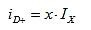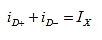?
EE Times-Asia > T&M
?
?
T&M??# Isolated potentiometer design (Part 2)

Posted: 22 Feb 2016 ?? ?Print Version ?

Keywords:isopot? op-amp? LM13700? BJTs? diodes?

Applying this algebraic identity to the above circuit equations,where iX = iD+ 鈭? iD-. This can also be expressed as a current-gain transfer function;The translinear cell is a linear differential-input, differential-output current amplifier. Because the static current ratios, IX and IY set the current gain, by varying either of them, the gain is varied. The diff-amp stage output current, through the unity-current-gain mirrors, is the amplifier output current, and the amplifier gain is given as the diff-amp stage gain above. It is inverting because the diodes are common-anode. (An alternative common-cathode connection, with anodes connected to the diff-amp bases, has positive gain.) The LM13700 can be used as a two-quadrant multiplier or VGA. The input circuit is shown below.This amplifier inputs a unipolar IY and a unipolar (positive) vI . When speed is a consideration, it is best to make vI the gain control and IY (which becomes iY ) the faster waveform. This implementation has a voltage-source input as shown below. When vI = 0 V, then the two sides of the circuit are symmetrical and iD- = iD+ = IX/2.Let iX be the differential current,Translinear circuits are easier to analyse using the variable, x, to represent the fraction of current that is conducted by one side. Here letBy KCL,and thusThe range of x is ±1. However, to switch all the current from one diode to the other, an infinite differential voltage is required. Because x is a hyperbolic tangent function of vX, the current only asymptotically approaches a complete switchover. Huge voltages are theoretically required to switch decades of currents at near-infinitesimal values. In practice, only about ±150 mV will switch a differential BJT or diode pair.

Translinear circuit design
Translinear circuit design requires that a design decision be made about the full-scale value of x. The zero-scale value is x = 0.5, where iD+ = iD- . For a choice of x(fs) = 0.75, then the ratio of diode currents isand ln(3) 鈮? 1.009 鈮? 1. The output fraction, iO /IY at full-scale is also 0.5, and the full-scale multiplier (or VGA) gain is 0.5. Therefore, IY must be twice as large as the desired iO.

Substituting into the previously derived equation for vI and applying a KVL equation involving the resistors,?
?
WebinarsVisit Asia Webinars to learn about the latest in technology and get practical design tips.

?
?